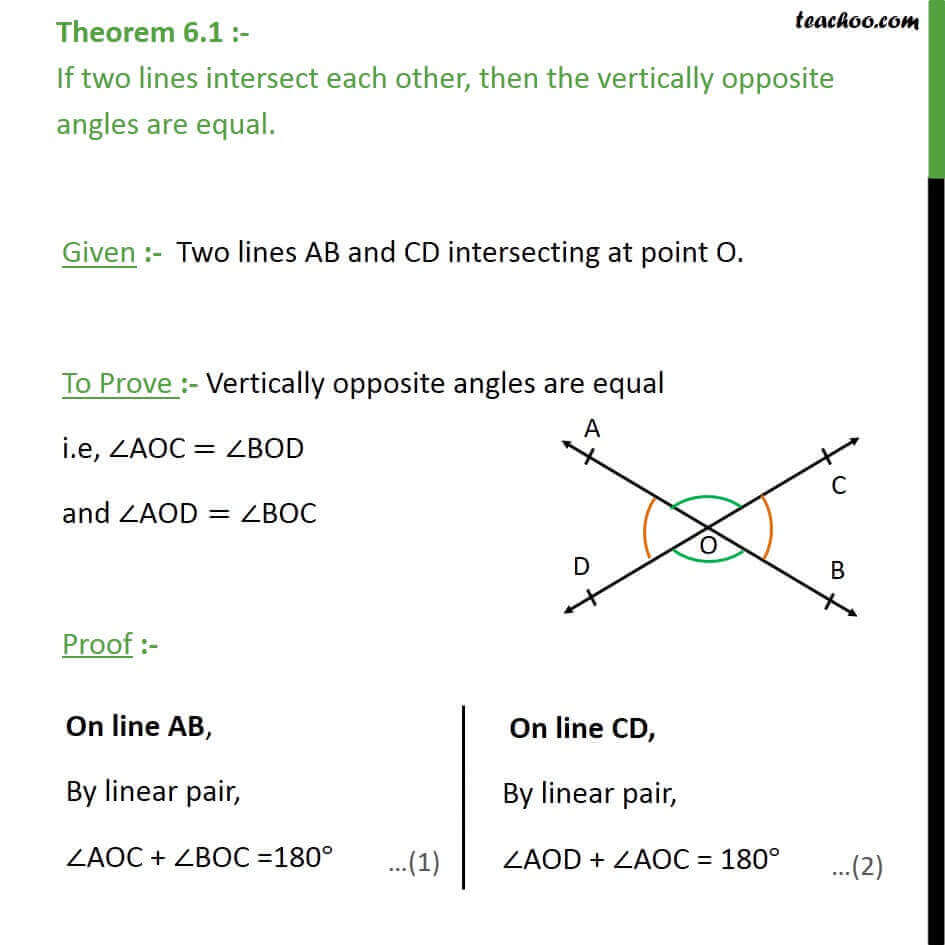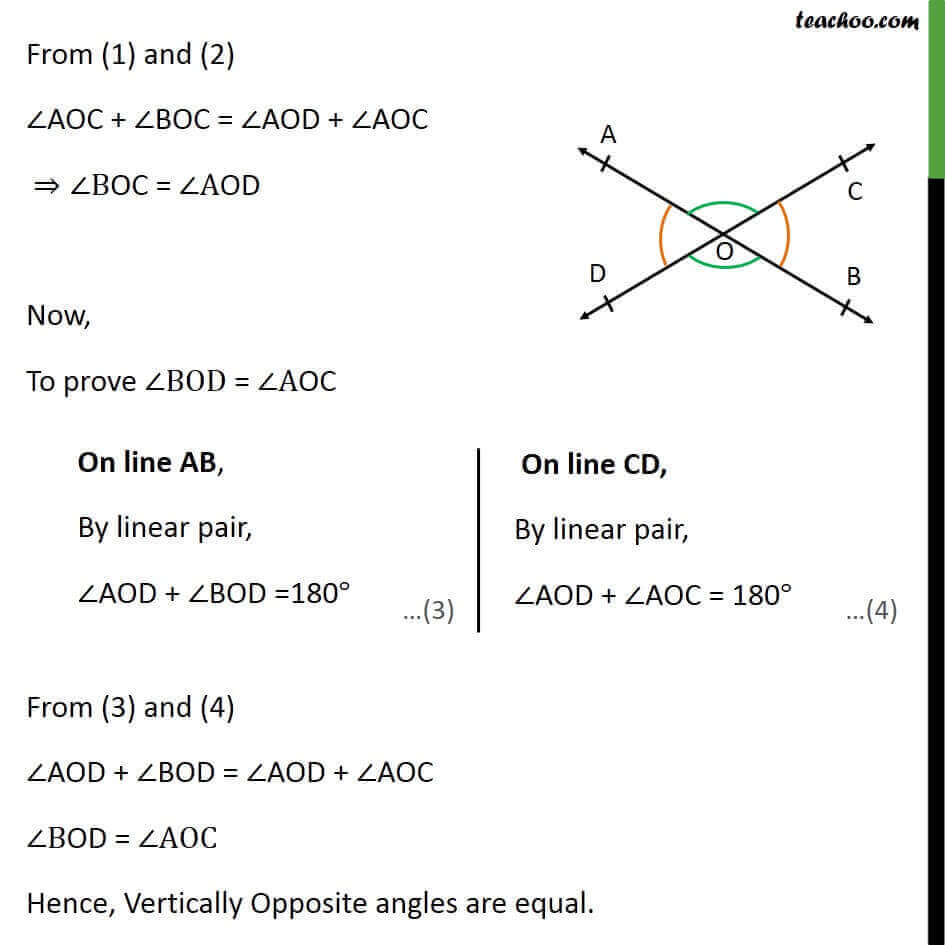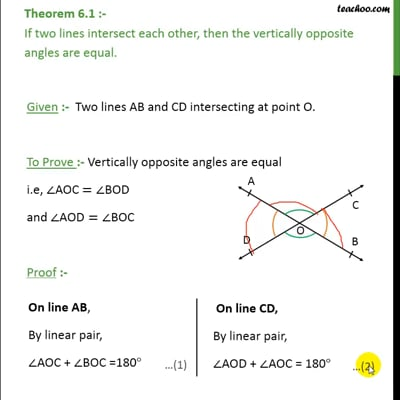Theorems

Chapter 6 Class 9 Lines and Angles (Term 1)
Serial order wiseThis video is only available for Teachoo black users

### Transcript

Theorem 6.1 :- If two lines intersect each other, then the vertically opposite angles are equal. Given :- Two lines AB and CD intersecting at point O. To Prove :- Vertically opposite angles are equal i.e, AOC = BOD and AOD= BOC Proof :- From (1) and (2) AOC + BOC = AOD + AOC BOC = AOD Now, To prove BOD = AOC From (3) and (4) AOD + BOD = AOD + AOC BOD = AOC Hence, Vertically Opposite angles are equal.